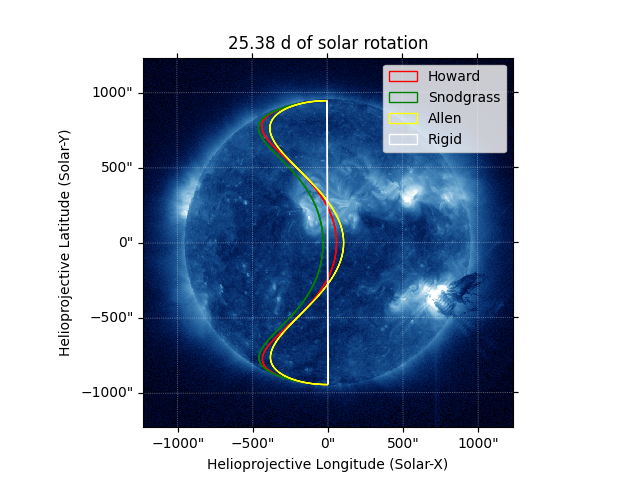# Comparing differential-rotation models#

How to compare differential-rotation models.

The example uses the RotatedSunFrame coordinate metaframe in sunpy.coordinates to apply differential rotation to a coordinate. See Differential rotation using coordinate frames for more details on using RotatedSunFrame.

import matplotlib.pyplot as plt

import astropy.units as u
from astropy.coordinates import SkyCoord

import sunpy.map
from sunpy.coordinates import HeliographicStonyhurst, RotatedSunFrame
from sunpy.data.sample import AIA_335_IMAGE
from sunpy.sun.constants import sidereal_rotation_rate


First, we use an AIA observation primarily as a pretty background. We also define the meridian using a two-element coordinate array of the south pole and the north pole at zero longitude.

aiamap = sunpy.map.Map(AIA_335_IMAGE)
meridian = SkyCoord(0*u.deg, [-90, 90]*u.deg, frame=HeliographicStonyhurst,
obstime=aiamap.date)


Next, we calculate the sidereal rotation period of the Sun. This is the time for a full rotation relative to an inertial reference frame (e.g., distant stars), as opposed to the synodic period, which is the apparent rotation period as seen from an Earth-based observer. Since the Earth orbits the Sun in the same direction as the Sun rotates, the Sun appears to rotate slower for an Earth-based observer.

sidereal_period = 360*u.deg / sidereal_rotation_rate
print(sidereal_period)

25.379994924001014 d


We use RotatedSunFrame to rotate the meridian by one sidereal period using each of the available differential-rotation models. See diff_rot() for details on each model.

rotated_meridian = {}
for model in ['howard', 'snodgrass', 'allen', 'rigid']:
rotated_meridian[model] = SkyCoord(RotatedSunFrame(base=meridian,
duration=sidereal_period,
rotation_model=model))


Finally, we plot the differentially rotated meridians over the map, using draw_quadrangle() to conveniently draw a line of constant longitude in the original frame between two endpoints. (One could instead use astropy.visualization.wcsaxes.WCSAxes.plot_coord(), but then meridian would need to consist of a sequence of many points spanning all latitudes between the two poles to render as desired.) Note that the “rigid” model appears as the meridian again as expected for a rotation of exactly one sidereal period.

fig = plt.figure()
aiamap.plot(axes=ax, clip_interval=(0.5, 99.9)*u.percent)

colors = {
'howard': 'red',
'snodgrass': 'green',
'allen': 'yellow',
'rigid': 'white',
}
for model, coord in rotated_meridian.items():